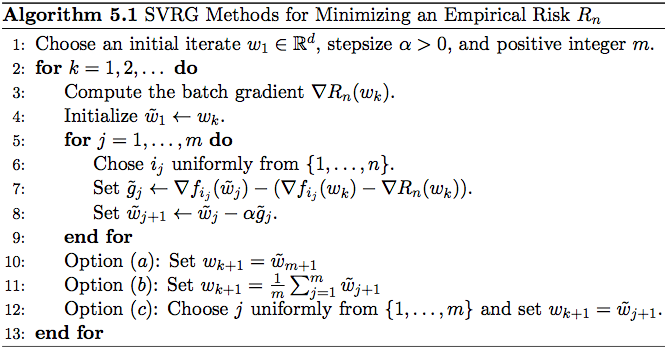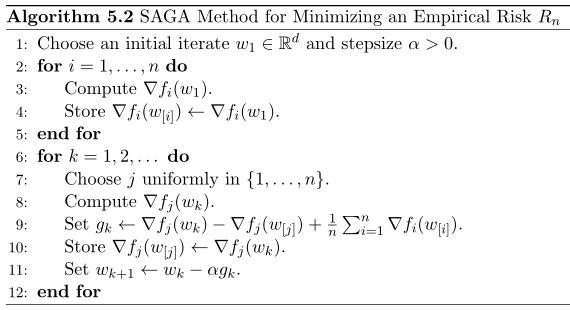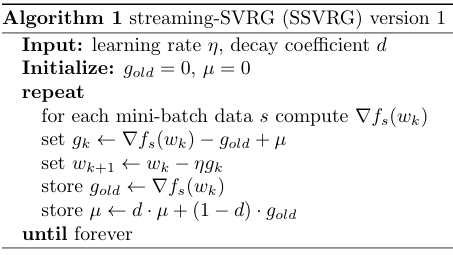# SVRG算法的阅读理解和实践

2018-05-11
caoxiaoqing

## 1. 背景介绍

$min \, R_n(w)=\frac{1}{n}\sum_{i=1}^{n}f_i(w)$

$w_{k+1}=w_{k}-\eta_k\nabla R_n(w_{k})=w_{k}-\frac{\eta_k}{n}\sum_{i=1}^{n}\nabla f_{i}(w_{k})$

$w_{k+1}=w_{k}-\eta_k\nabla f_{i_k}(w_{k})$

## 2. 方差缩减方法 Noise Reduction Methods

$\theta_{\alpha}=\alpha(X-Y)+\mathbb{E}Y$

$\mathbb{E}\theta_{\alpha}=\alpha\mathbb{E}X+(1-\alpha)\mathbb{E}Y \\ Var(\theta_{\alpha})=\alpha^2[Var(X)+Var(Y)-2Cov(X,Y)]$

## 3. SAG 算法 

SAG 算法想啊，既然 GD 用上所有数据的梯度就能做到线性收敛，SGD 一次只能用一个样本或者一小批样本来计算梯度，因此对梯度估计的方差影响了最终的收敛速度，那我们能不能在 SGD 上也能用上所有样本的梯度呢？于是就有了 SAG 算法的更新方式：

w_{k+1}=w_k-\frac{\eta_k}{n}\sum_{i=1}^{n}y_i^{k} \\ y_i^{k}=\left\{ \begin{aligned} &\nabla f_i(w_k), \, &\text{if}\,i_k=i\\ &\nabla f_i(w_{k-1}) \, &\text{otherwise} \end{aligned} \right.

$\tilde g_k \, \leftarrow \, \frac{\nabla f_{i_k}(w_k) - \nabla f_{i_k}(w_{k-1})}{n} + \nabla R_n(w_{k-1})$

## 4. SVRG 算法 

SVRG 算法的迭代过程 (图片来自文章 )SVRG 算法在每一轮迭代的内部有一个内部的迭代，在进行内部迭代前用当前的 $w_k$ 值计算一次所有样本的平均梯度 $\nabla R_n(w_k)$，内部迭代的初始值被赋予为当前的 $w_k$，内部迭代中每次的梯度采用如下方式计算：

$\tilde g_j \, \leftarrow \, \nabla f_{i_j}(\tilde w_j)-(\nabla f_{i_j}(w_k)-\nabla R_n(w_k))$

SVRG 算法的问题是虽然不用存储所有样本的梯度了，但是计算量上去了，因为它在每次的大迭代里面还有一轮小迭代，每次都要算两遍梯度，整体的计算量已经和 GD 一样了，而且还多一个超参数 $m$ 要调。另外，这里 有一个 PPT ，讲的还比较通俗，可以随意看看。

## 5. SAGA 算法 

SAGA 算法其实是介于 SAG 和 SVRG 之间的一种算法，但作者声称在强凸的条件下其收敛速度要快于 SAG 和 SVRG，且两倍于 SDCA，并同时适用于非强凸的情形。

SAGA 算法的迭代过程 (图片来自文章 )$\tilde g_k \, \leftarrow \, \nabla f_{i_k}(w_k) - \nabla f_{i_k}(w_{k-1}) + \nabla R_n(w_{k-1})$

## 6. SCSG 算法 

SVRG 的主要特征就是利用全部数据的梯度来对 SGD 的方差进行控制。因此 SVRG 的计算成本（Computation Cost）是 $O((n+m)T)$。这里 $n$ 是数据的总数，$m$ 是 Step-size，而 $T$ 是论数。SVRG 的通讯成本也是这么多，这里面的主要成本在于每一轮都需要对全局数据进行访问。

Stochastically Controlled Stochastic Gradient（SCSG）算法就是对 SVRG 进行了两个改进：

• 每一轮并不用全局的数据进行梯度的计算，而是从一个全局的子集 Batch 中估计梯度，子集的大小是 B。
• 每一轮 SGD 的更新数目 $N$ 也不是一个定值，而是一个和之前那个子集大小有关系，基于 Geometric Distribution 的随机数。

## 7. 其它方法

Mini-Batch Semi-Stochastic Gradient Descent in the Proximal Setting. 将S2GD扩展到mini-batch上，因此允许并行运行，但是需要更多的同步，只能允许小的batch

SDCA(Stochastic dual coordinate ascent methods for regularized loss) 

Finito(Finito: A faster, permutable incremental gradientmethod for big data problems)

## 8. 应用于在线学习• $g_{old}$ 来自上一次的梯度，这里可以不要求是同一个 mini batch 的数据，其中的 $w_k$ 也可以是被其他 worker 更新后的值，因此，这个算法可以适用于多 worker 异步更新的场合，因为我们可以假设在很短的一个时间内，所有 worker 拿到的数据都是独立同分布的。
• 为了获得上文第二部分所说的 $\mathbb{E}Y$，也就是上文其他算法中的 $\nabla R_n(w_k)$，我们使用了一个滑动加权平均的方式，其中参数 $d$ 是为了控制历史值与当前值的权重分配。

## Reference

 Cauchy, Augustin. “Méthode générale pour la résolution des systemes d’équations simultanées.” Comp. Rend. Sci. Paris 25.1847 (1847): 536-538.

 Robbins, Herbert, and Sutton Monro. “A stochastic approximation method.” The annals of mathematical statistics (1951): 400-407.

 Kiefer, Jack, and Jacob Wolfowitz. “Stochastic estimation of the maximum of a regression function.” The Annals of Mathematical Statistics 23.3 (1952): 462-466.

 Bottou, Léon, Frank E. Curtis, and Jorge Nocedal. “Optimization methods for large-scale machine learning.” SIAM Review 60.2 (2018): 223-311.

 Johnson, Rie, and Tong Zhang. “Accelerating stochastic gradient descent using predictive variance reduction.” Advances in Neural Information Processing Systems. 2013.

 Defazio, Aaron, Francis Bach, and Simon Lacoste-Julien. “Saga: A fast incremental gradient method with support for non-strongly convex composite objectives.” Advances in Neural Information Processing Systems. 2014.

 Shalev-Shwartz, Shai, and Tong Zhang. “Accelerated mini-batch stochastic dual coordinate ascent.” Advances in Neural Information Processing Systems. 2013.

 Lei, Lihua, and Michael Jordan. “Less than a Single Pass: Stochastically Controlled Stochastic Gradient.” Artificial Intelligence and Statistics. 2017.

 Nitanda, Atsushi. “Stochastic proximal gradient descent with acceleration techniques.” Advances in Neural Information Processing Systems. 2014.

 Roux, Nicolas L., Mark Schmidt, and Francis R. Bach. “A stochastic gradient method with an exponential convergence _rate for finite training sets.” Advances in Neural Information Processing Systems. 2012.